# subtitle

## Syntax

subtitle(txt)
subtitle(___,Name,Value)
subtitle(target,___)
t = subtitle(___)

## Description

example

subtitle(txt) adds the specified subtitle text to the current axes.

example

subtitle(___,Name,Value) sets properties on the text object using one or more name-value pair arguments. Specify the properties after all other input arguments. For a list of properties, see Text Properties.

example

subtitle(target,___) specifies the target object for the subtitle. The target object can be any type of axes, a tiled chart layout, or an array of objects. Specify the target object before all other input arguments.

example

t = subtitle(___) returns the text object for the subtitle. Use t to set properties on the object after creating the subtitle. For a list of properties, see Text Properties.

## Examples

collapse all

Create a plot. Add a title with the title function. Then add a subtitle with the subtitle function.

plot([0 2],[1 5]) title('Straight Line') subtitle('Slope = 2, y-Intercept = 1')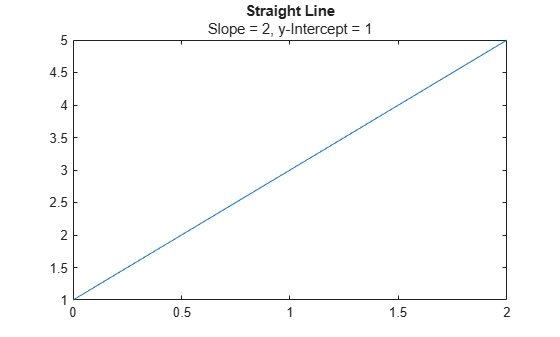Create a plot, and add a title to the plot. Define slopevalue and yintercept as numeric variables. Define txt as a combination of literal text and the values of slopevalue and yintercept converted to character vectors. Then, pass txt to the subtitle function to display the subtitle.

plot([0 2],[1 5]) title('Straight Line') slopevalue = 4; yintercept = 1; txt = ['Slope = ' int2str(slopevalue) ', y-Intercept = ' int2str(yintercept)]; subtitle(txt)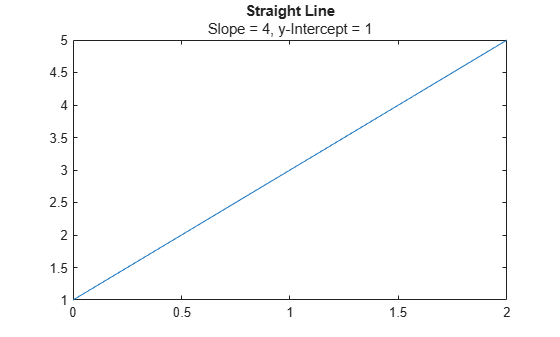Create a plot. Add a title with the title function. Then, call the subtitle function, and specify the color using the 'Color' name-value pair argument. The color can be a color name, such as 'red', or you can specify a custom color using an RGB triplet or hexadecimal color code. In this case, specify 'red'.

plot([0 2],[1 5]) title('Straight Line') subtitle('Slope = 2, y-Intercept = 1','Color','red')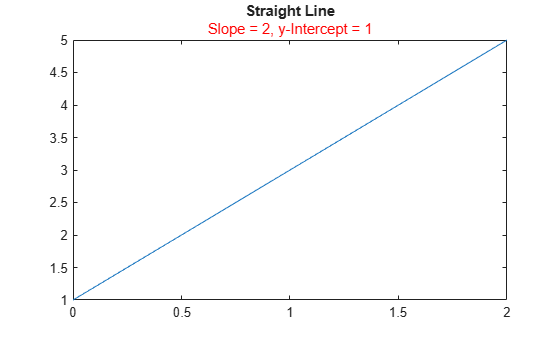Alternatively, call the subtitle function with an output argument to return the text object. Then set the color on the text object. In this case, specify the hexadecimal color code '#DD5500'.

txt = subtitle('Plot of y = 2x + 1'); txt.Color = '#DD5500';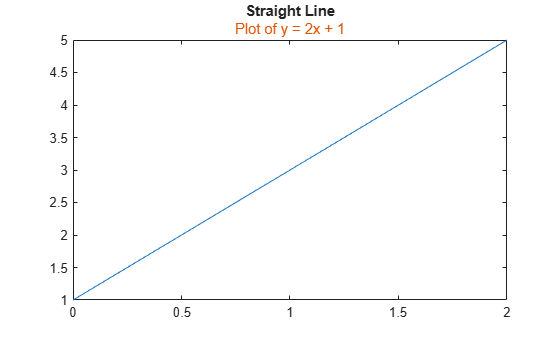Create a plot, and add a title with the title function. Create a character vector containing TeX markup with custom colors for different words in the subtitle. Then pass the character vector to the subtitle function.

plot([0 2],[1 5]) title('Straight Line') txt = ['An {\color{magenta}Attractive '... '\color[rgb]{0 .5 .5}and \color{red}Colorful} Subtitle']; subtitle(txt)Create a histogram, and add a title with the title function. Create a character vector containing TeX markup with Greek symbols. Then pass the character vector to the subtitle function.

histogram(5*randn(1,50)+10) title('Population Data') txt = '{\it\mu} = 10, {\it\sigma} = 5'; subtitle(txt)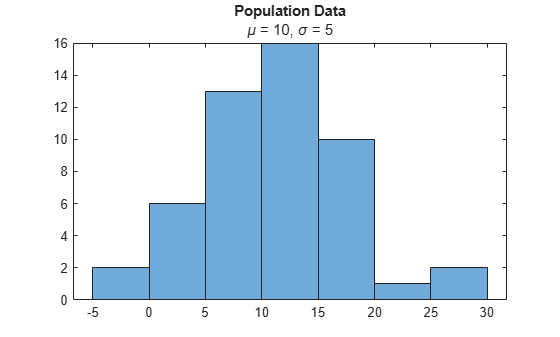Create a histogram, and add a title with the title function. Create a character vector containing TeX markup that displays subscripts and superscripts. Then pass the character vector to the subtitle function.

x = -10:0.1:10; y1 = x.^2; y2 = 2*x.^2; plot(x,y1,x,y2); title('Exponential Functions') txt = 'y_1 = x^2 and y_2 = 2x^{2 + k}'; subtitle(txt)To display an italic font for the variables, add the \it modifier.

txt = '{\ity}_1 = {\itx}^2 and {\ity}_2 = 2{\itx}^{2 + \itk}'; subtitle(txt)Create a plot, and add a title with the title function. Then create a subtitle containing two lines of text by passing a cell array of character vectors to the subtitle function. Each element in the array is a separate line of text.

plot([0 2],[1 5]) title('Straight Line') txt = {'Slope = 2','y-Intercept = 1'}; subtitle(txt)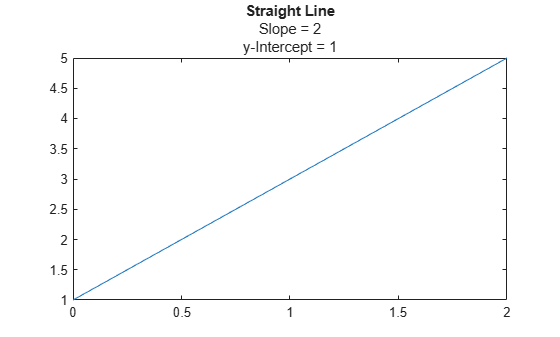Create a plot with a title. Then create a subtitle containing an underscore character that the TeX interpreter normally uses for subscripts. Set the Interpreter to 'none' when you call the subtitle function, so that the underscore character appears in the subtitle.

plot([0 2],[1 5]) title('Straight Line') subtitle('y_1 = 2x + 1','Interpreter','none')Create a plot and add a title and a subtitle. Get the current axes, and align the title and subtitle to the left edge of the plot box by setting the TitleHorizontalAlignment property on the axes to 'left'.

plot([0 2],[1 5]) title('Straight Line') subtitle('Slope = 2, y-Intercept = 1') ax = gca; ax.TitleHorizontalAlignment = 'left';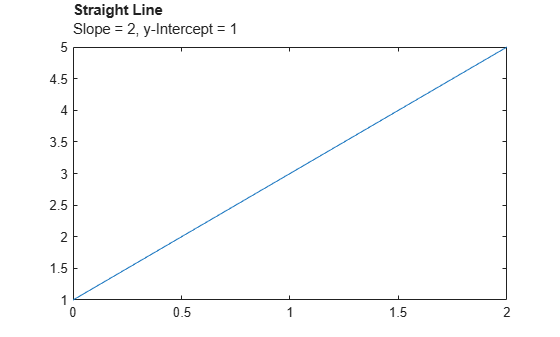Center the title and subtitle by setting the TitleHorizontalAlignment property on the axes to 'center'.

ax.TitleHorizontalAlignment = 'center';Create two plots in a tiled chart layout. Then add a title and subtitle to each plot.

t = tiledlayout(1,2); % Left plot ax1 = nexttile; plot([0 2],[1 5]) title(ax1,'A Straight Line') subtitle(ax1,'Slope = 2, y-Intercept = 1') % Right plot ax2 = nexttile; plot([0 2],[2 8]) title(ax2,'Another Straight Line') subtitle(ax2,'Slope = 3, y-Intercept = 2')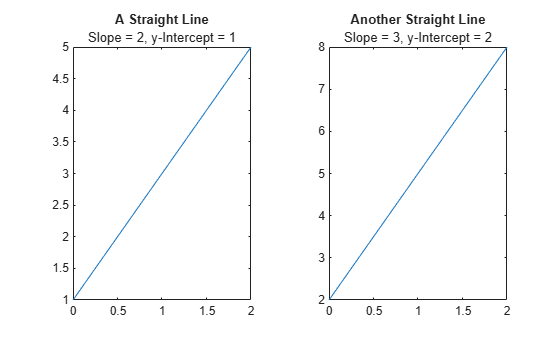## Input Arguments

collapse all

Subtitle text, specified as a character vector, cell array of character vectors, or a string array. To create multiple lines of text, specify a cell array of character vectors or a string array.

Example: subtitle('Single Line Subtitle')

Example: subtitle(["Subtitle With" "Multiple Lines"])

Target for the subtitle, specified as one of the following:

• Any type of axes: an Axes, PolarAxes, or GeographicAxes object.

• A TiledChartLayout object.

• An array of graphics objects from the preceding list. The objects must belong to the same class. To determine the class, use the class function.

If you do not specify the target for the subtitle, then the subtitle function adds the subtitle to the graphics object returned by the gca command.

### Name-Value Arguments

Specify optional pairs of arguments as Name1=Value1,...,NameN=ValueN, where Name is the argument name and Value is the corresponding value. Name-value arguments must appear after other arguments, but the order of the pairs does not matter.

Before R2021a, use commas to separate each name and value, and enclose Name in quotes.

Example: subtitle('My Subtitle','FontSize',12) specifies a 12-point font size.

Note

The properties listed here are only a subset. For a complete list, see Text Properties.

Font size, specified as a scalar value greater than 0 in point units. One point equals 1/72 inch. To change the font units, use the FontUnits property.

If you add a title or subtitle to an axes object, then the font size property for the axes also affects the font size for the title and subtitle. The title and subtitle font sizes are the axes font size multiplied by a scale factor. The FontSize property of the axes contains the axes font size. The TitleFontSizeMultiplier property of the axes contains the scale factor. By default, the axes font size is 10 points and the scale factor is 1.1, so the title and subtitle each have a font size of 11 points.

Data Types: single | double | int8 | int16 | int32 | int64 | uint8 | uint16 | uint32 | uint64

Thickness of the text characters, specified as one of these values:

• 'normal' — Normal weight as defined by the particular font

• 'bold' — Thicker characters outlines than normal

MATLAB® uses the FontWeight property to select a font from those available on your system. Not all fonts have a bold font weight. Therefore, specifying a bold font weight could still result in the normal font weight.

The SubtitleFontWeight property for the associated axes affects the FontWeight value for the subtitle.

## Tips

• By default, the Interactions property contains editInteraction so the text can be edited by clicking on the text. To disable this interaction, set the Interactions property of the text object to [].

## Version History

Introduced in R2020b